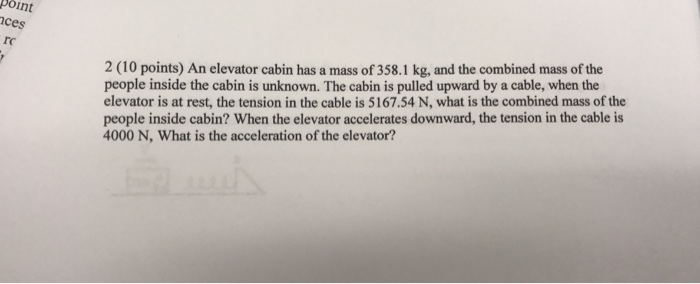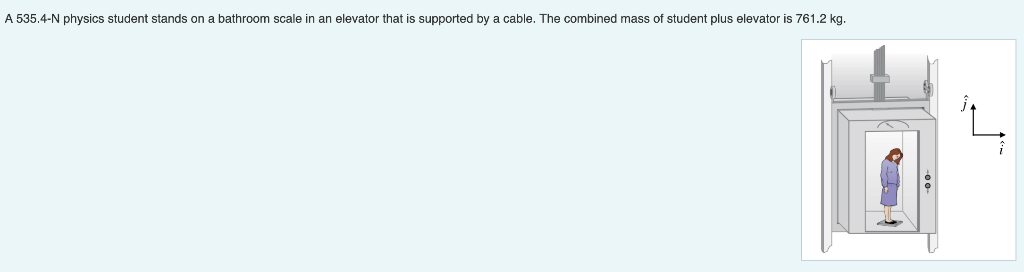Question

# A woman stands on a scale in a moving elevator. Her mass is 55.0 kg, and...

A woman stands on a scale in a moving elevator. Her mass is 55.0 kg, and the combined mass of the elevator and scale is an additional 797 kg. Starting from rest, the elevator accelerates upward. During the acceleration, the hoisting cable applies a force of 9190 N. What does the scale read (in N) during the acceleration? Number Units

Balancing the forces,

(797 + 55) a = 9190 - (797 + 55) x 9.8

Acceleration, a = 0.986 m/s^2

Weight, W = 55 x (9.8 + 0.986) = 593.25 N

#### Earn Coins

Coins can be redeemed for fabulous gifts.

Similar Homework Help Questions
• ### A woman stands on a scale in a moving elevator. Her mass is 62.0 kg, and...

A woman stands on a scale in a moving elevator. Her mass is 62.0 kg, and the combined mass of the elevator and scale is an additional 838 kg. Starting from rest, the elevator accelerates upward. During the acceleration, the hoisting cable applies a force of 9140 N. What does the scale read (in N) during the acceleration?

• ### A woman stands on a scale in a moving elevator. Her mass is 62.0 kg, and...

A woman stands on a scale in a moving elevator. Her mass is 62.0 kg, and the combined mass of the elevator and scale is an additional 772 kg. Starting from rest, the elevator accelerates upward. During the acceleration, the hoisting cable applies a force of 9930 N. What does the scale read (in N) during the acceleration?

• ### A woman stands on a scale in a moving elevator. Her mass is 57.5 kg, and...

A woman stands on a scale in a moving elevator. Her mass is 57.5 kg, and the combined mass of the elevator and scale is an additional 815 kg. Starting from rest, the elevator accelerates upward. During the acceleration, the hoisting cable applies a force of 9870 N. What does the scale read during the acceleration?

• ### Chapter 4 Problem 42 A woman stands on a scale in a moving elevator. Her mass...

Chapter 4 Problem 42 A woman stands on a scale in a moving elevator. Her mass is 63.0 kg, and the combined mass of the elevator and scale is an additional 790 kg. Starting from rest, the elevator accelerates upward. During the acceleration, the hoisting cable applies a force of 9130 N. What does the scale read (in N) during the acceleration?

• ### An elevator cabin has a mass of 358.1 kg, and the combined mass of the people...An elevator cabin has a mass of 358.1 kg, and the combined mass of the people inside the cabin is unknown. The cabin is pulled upward by a cable, when the elevator is at rest, the tension in the cable is 5167.54 N, what is the combined mass of the people inside cabin? When the elevator accelerates downward, the tension in the cable is 4000 N, What is the acceleration of the elevator?

• ### A 535.4-N physics student stands on a bathroom scale in an elevator that is supported by...A 535.4-N physics student stands on a bathroom scale in an elevator that is supported by a cable. The combined mass of student plus elevator is 761.2 kg Part A What is the acceleration if the scale reads 416 N as the elevator starts moving? Notice that g 9.8 m/s2 and the sign of the acceleration is determined by the direction. For example, the gravitational acceleration is -g--9.8m/s as the gravitational force points downward m's Submit Request Answer Part B...

• ### Henry, whose mass is 95 kg, stands on a bathroom scale in an elevator. The scale...

Henry, whose mass is 95 kg, stands on a bathroom scale in an elevator. The scale reads 830 N for the first 2.8 s after the elevator starts moving, then 930 N for the next 2.8 s. What is the elevator's speed 5.6 s after starting?

• ### A 72 kg man stands on a bathroom scale in an elevator. Starting from rest at...

A 72 kg man stands on a bathroom scale in an elevator. Starting from rest at the top floor, the elevator descends, attaining its maximum speed of 1.20 m/s in 0.80 s. It travels with this constant speed for the next 5.00 s. The elevator then slows down for 1.50 s and comes to rest at ground level. a)What is the acceleration of the man during the first 0.80 s? Answer in units of m/s2 and use the upward direction...

• ### A 68-kg man stands on a spring scale in an elevator. Starting from rest, the elevator...

A 68-kg man stands on a spring scale in an elevator. Starting from rest, the elevator ascends, attaining its maximum speed of 1.2 m/s in 0.77 s. The elevator travels with this constant speed for 5.0 s, undergoes a uniform negative acceleration for 1.8 s, and then comes to rest. (a) What does the spring scale register before the elevator starts to move? N (b) What does the spring scale register during the first 0.77 s of the elevator's ascent?...

• ### A 596-N physics student stands on a bathroom scale in an 849-kg (including the student) elevator...

A 596-N physics student stands on a bathroom scale in an 849-kg (including the student) elevator that is supported by a cable. As the elevator starts moving, the scale reads 449 N. a)Find the magnitude of the acceleration of the elevator. b) What is the acceleration if the scale reads 659 N ? c) If the scale reads zero, should the student worry? Explain. d)What is the tension in the cable in part A? e)What is the tension in the...